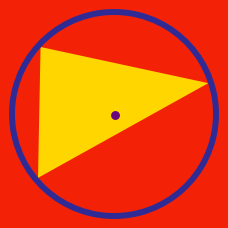Geometry

# Triangle Centers: Level 2 Challenges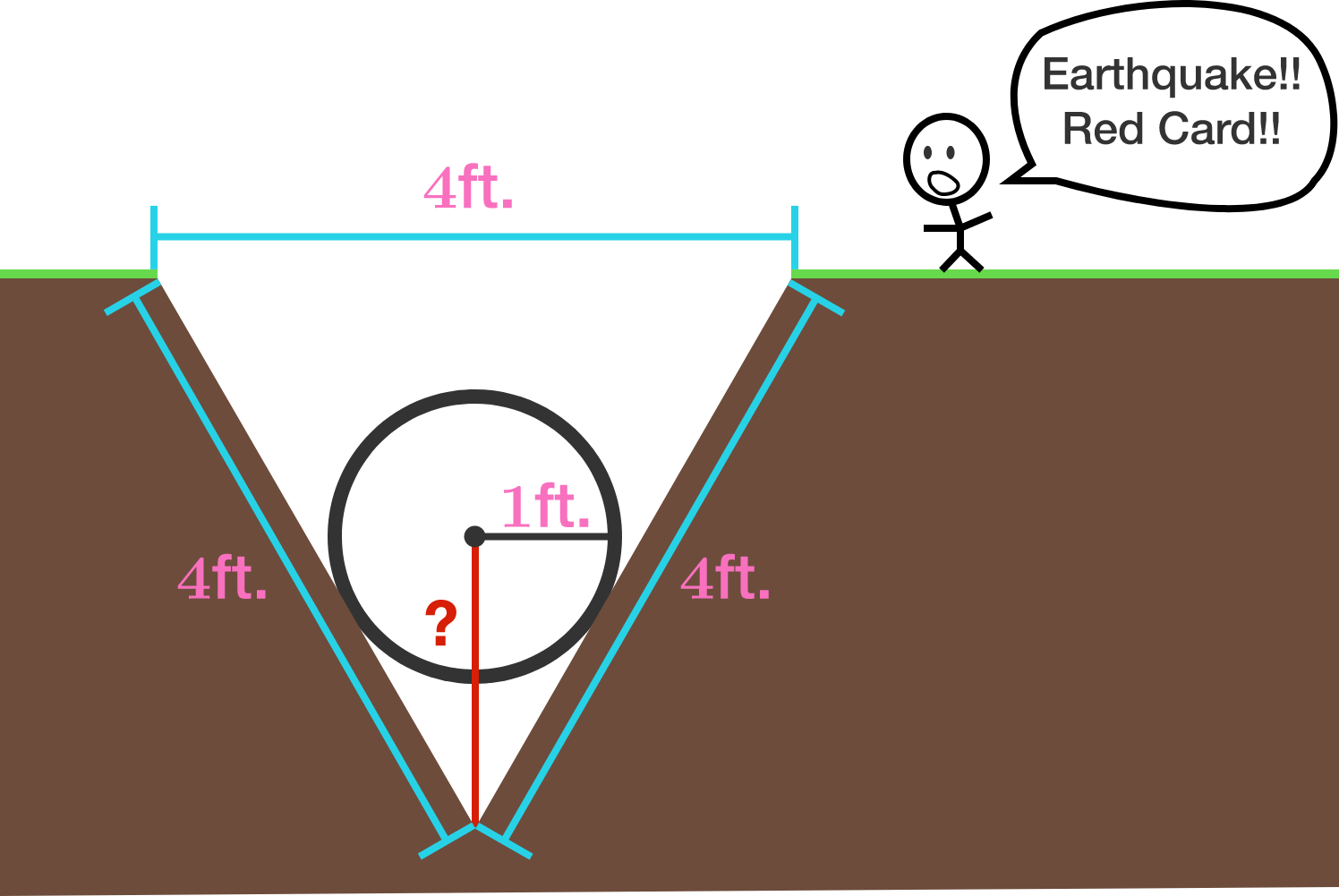At the 1920 FIFA World Cup, there was an earth quake during one of the games and a cone shaped hole was created with a diameter of 4 feet and sloping sides, both 4 feet long from ground-level to the bottom of the pit. The soccer ball, with a radius of one foot, fell into the hole. Find the distance from the center of the Soccer ball to the bottom of the pit in feet.

Assumptions:
- The sides of the hole are perfectly straight and smooth.
- The ball falls as far as it can with out changing shape.
- FYI, there wasn't actually an earthquake at the 1920 FIFA World Cup.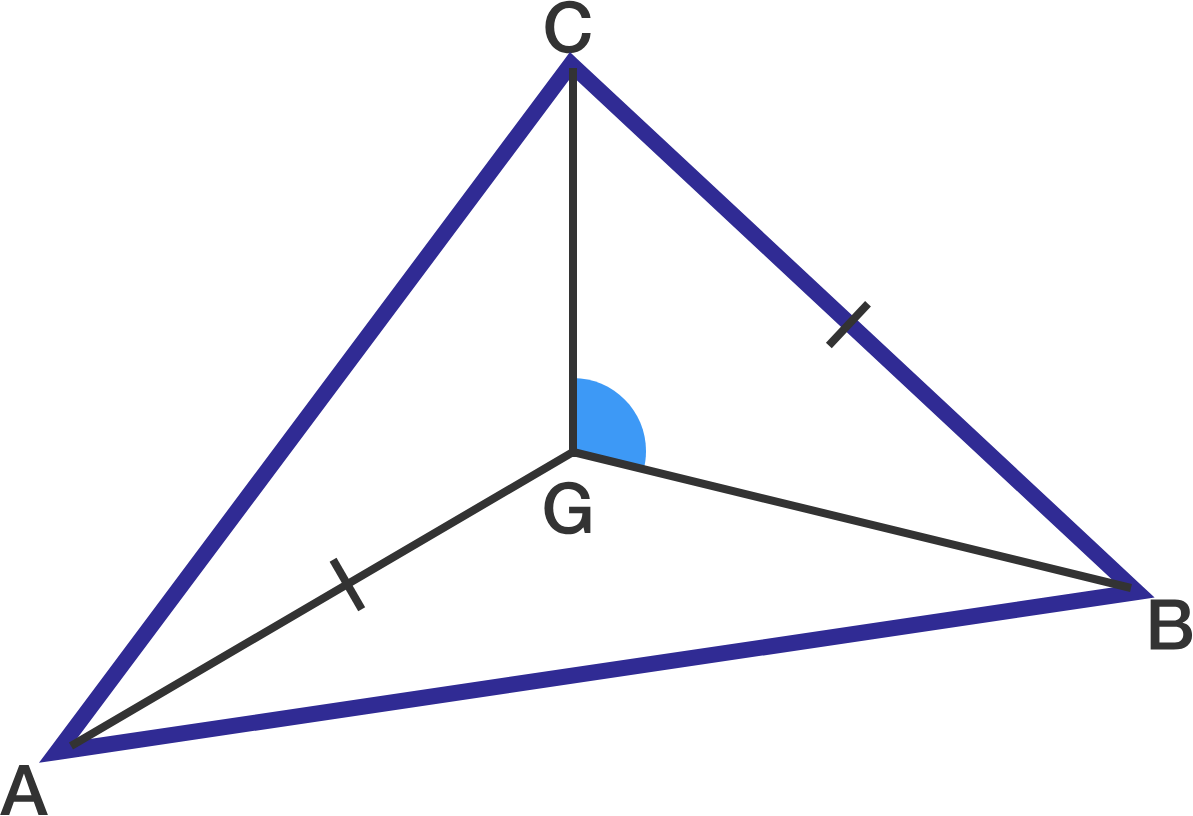In triangle $ABC$ with centroid $G$, if $AG=BC$, what is angle $BGC$ in degrees?

Note: Diagram is not drawn to scale.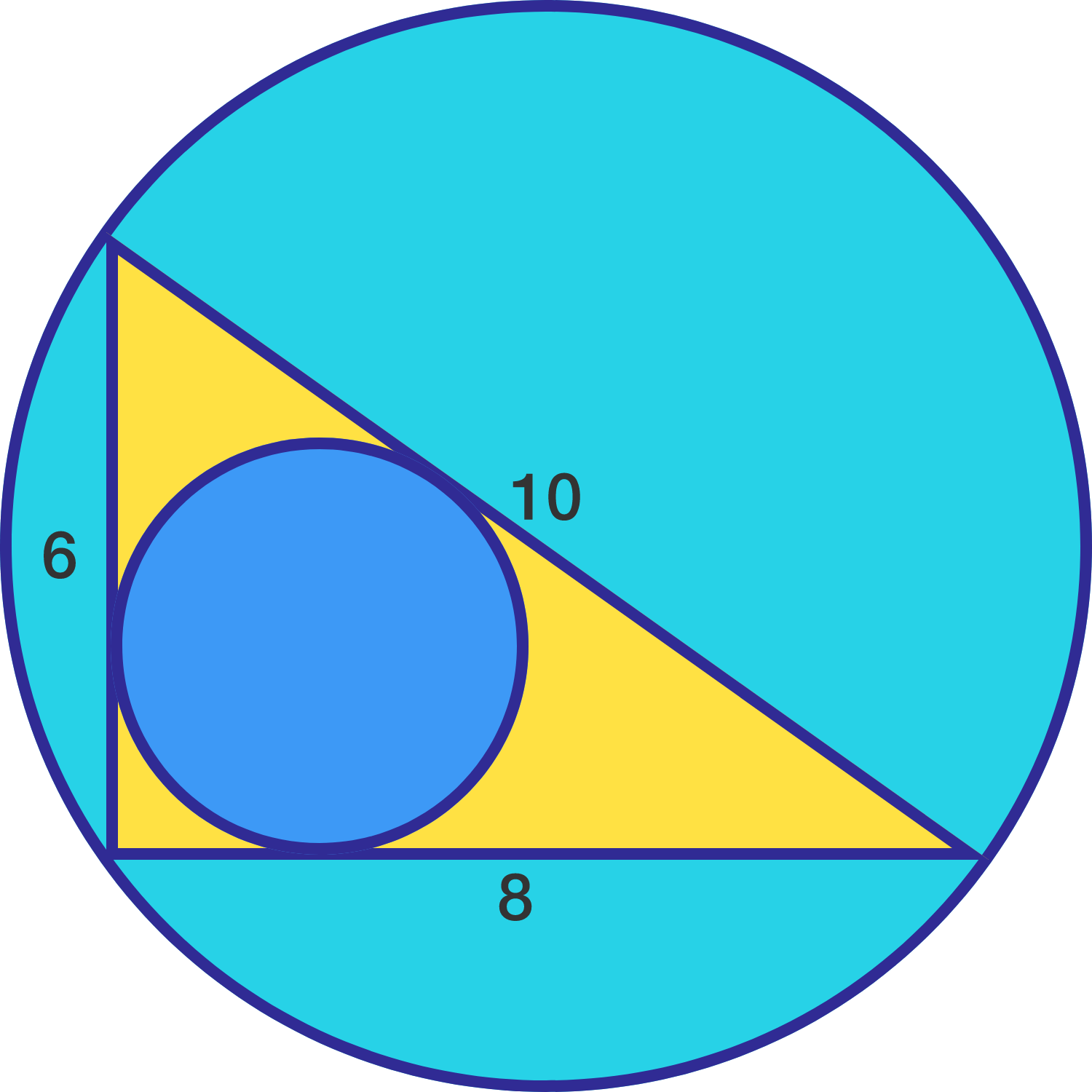A triangle has sides of 6, 8, and 10 inches.

What is the distance between incenter and circumcenter of the triangle?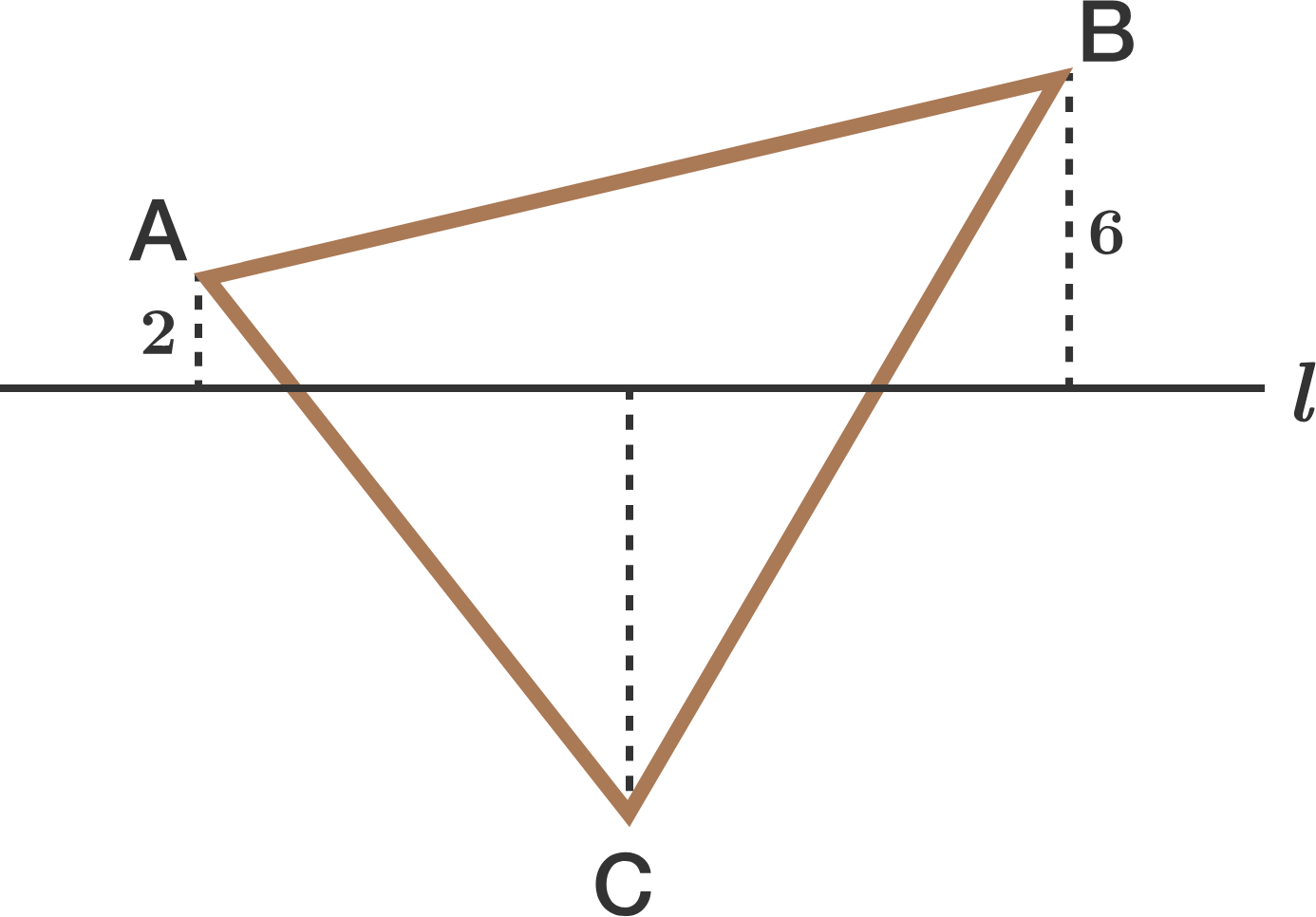In the diagram above, line $l$ passes through the centroid of $\triangle ABC.$

If the perpendicular distance between $A$ and line $l$ is 2, and the perpendicular distance between $B$ and line $l$ is 6, then what is the perpendicular distance between $C$ and line $l?$

Consider an isosceles $\triangle ABC$ with $AB=AC=5, BC=6,$ where $I,O,H$ denote its incenter, circumcenter, orthocenter, respectively.

Find the area of $\triangle IOH$.

×